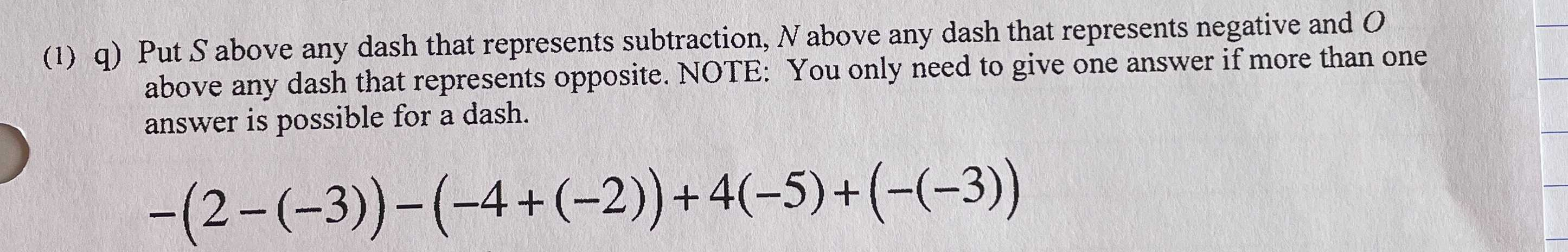### Still have math questions?

Algebra
Question(1) q) Put $$S$$ above any dash that represents subtraction, $$N$$ above any dash that represents negative and $$O$$ above any dash that represents opposite. NOTE: You only need to give one answer if more than one answer is possible for a dash.

$$- ( 2 - ( - 3 ) ) - ( - 4 + ( - 2 ) ) + 4 ( - 5 ) + ( - ( - 3 ) )$$